# 1 Water Usage of Production Plant

A production plant cost-control engineer is responsible for cost reduction. One of the costly items in his plant is the amount of water used by the production facilities each month. He decided to investigate water usage by collecting seventeen observations on his plant’s water usage and other variables.

Variable Description
Temperature Average monthly temperate (F)
Production Amount of production (M pounds)
Days Number of plant operating days in the month
Persons Number of persons on the monthly plant payroll
Water Monthly water usage (gallons)
library(tidyverse)

#   save in the same folder as this Rmd file.

str(dat_water)
tibble [17 x 5] (S3: spec_tbl_df/tbl_df/tbl/data.frame)
$Temperature: num [1:17] 58.8 65.2 70.9 77.4 79.3 81 71.9 63.9 54.5 39.5 ...$ Production : num [1:17] 7107 6373 6796 9208 14792 ...
$Days : num [1:17] 21 22 22 20 25 23 20 23 20 20 ...$ Persons    : num [1:17] 129 141 153 166 193 189 175 186 190 187 ...
\$ Water      : num [1:17] 3067 2828 2891 2994 3082 ...
- attr(*, "spec")=
.. cols(
..   Temperature = col_double(),
..   Production = col_double(),
..   Days = col_double(),
..   Persons = col_double(),
..   Water = col_double()
.. )
#dat_water

dat_water <-
dat_water %>%
mutate(
id = 1:n()
) %>%
# filter to remove observations (if needed)
#   TRUE        indicates that ALL observations are included
#   !(id %in% c(4, 12)) indicates to include all observations that are NOT id 4 or 12
filter(
TRUE  # !(id %in% c(4, 12))
)

Note: Because of the high correlation between Production and Persons, do not include Persons in the model.

# 2 Rubric

Following the in-class assignment this week, perform a complete multiple regression analysis.

1. (1 p) Scatterplot matrix and interpretation
2. (2 p) Fit model, assess multiple regression assumptions
3. (1 p) Interpret added variable plots
4. (1 p) If there are model assumption issues, say how you address them at the beginning and start again.
5. (1 p) State and interpret the multiple regression hypothesis tests
6. (2 p) Interpret the significant multiple regression coefficients
7. (1 p) Interpret the multiple regression $$R^2$$
8. (1 p) One- or two-sentence summary

# 3 Solutions

## 3.1(1 p) Scatterplot matrix

In a scatterplot matrix below interpret the relationship between each pair of variables. If a transformation is suggested by the plot (that is, because there is a curved relationship), also plot the data on the transformed scale and perform the following analysis on the transformed scale. Otherwise indicate that no transformation is necessary.

library(ggplot2)
library(GGally)
#p <- ggpairs(dat_water)
p <- ggpairs(dat_water %>% select(-id, -Persons))  ## use select to remove vars
print(p)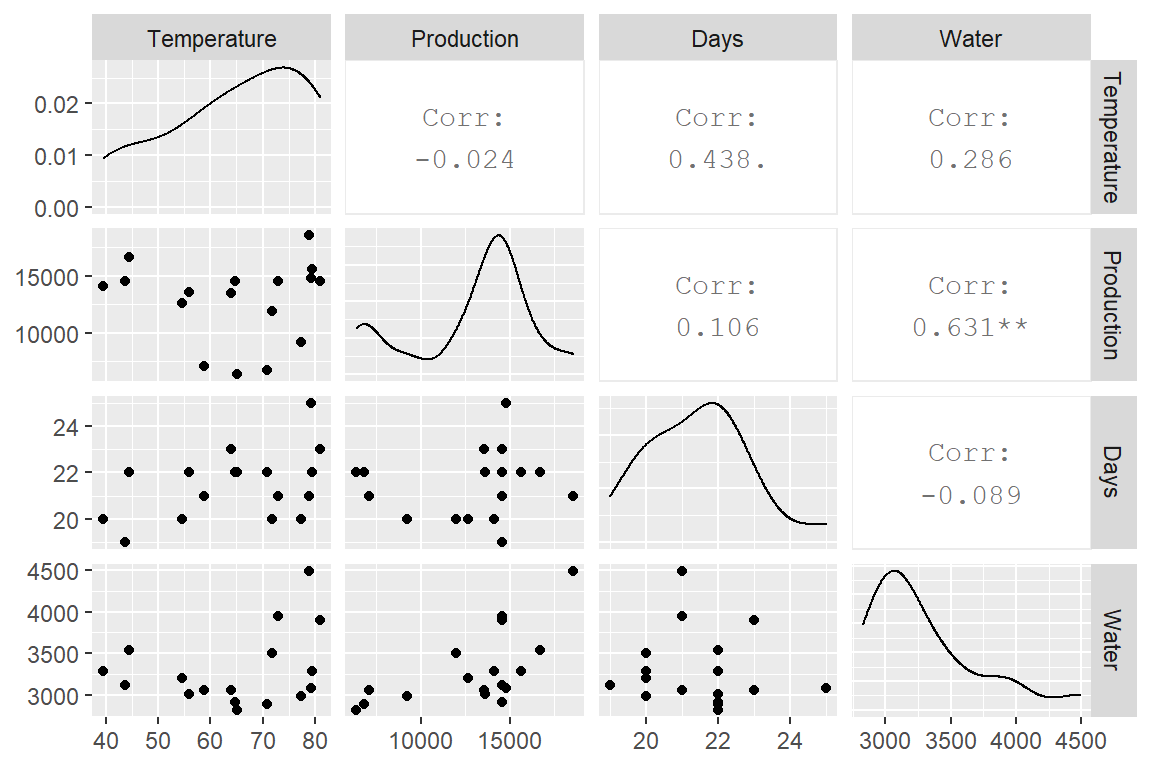A parallel coordinate plot is another way of seeing patterns of observations over a range of variables.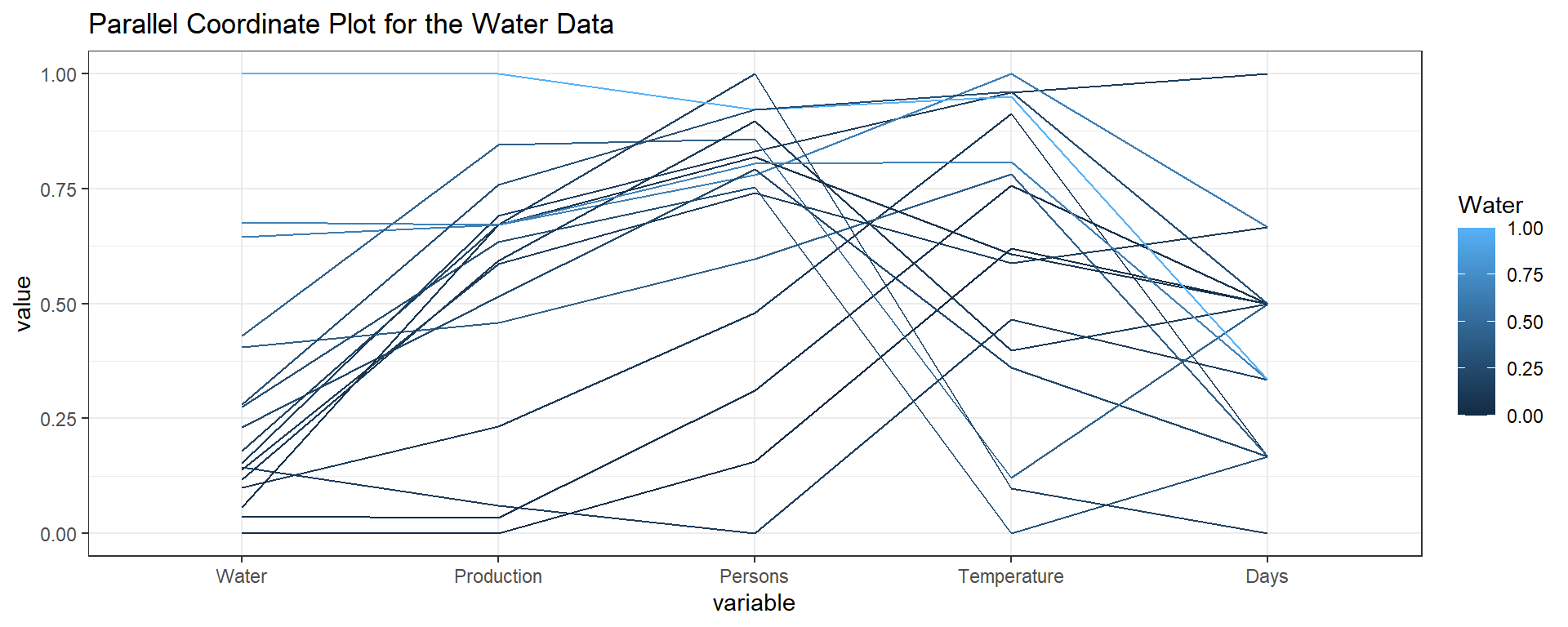## 3.2(2 p) Multiple regression assumptions (assessing model fit)

Below the multiple regression is fit. Start by assessing the model assumptions by interpretting what you learn from the first seven plots (save the added variable plots for the next question). If assumptions are not met, attempt to address by transforming a variable (or removing an outlier) and restart at the beginning using the new transformed variable.

# fit the simple linear regression model
#lm_w_tpdp <- lm(Water ~ Temperature + Production + Days + Persons, data = dat_water)
lm_w_tpd <-
lm(
Water ~ Temperature + Production + Days
, data = dat_water
)

Plot diagnostics.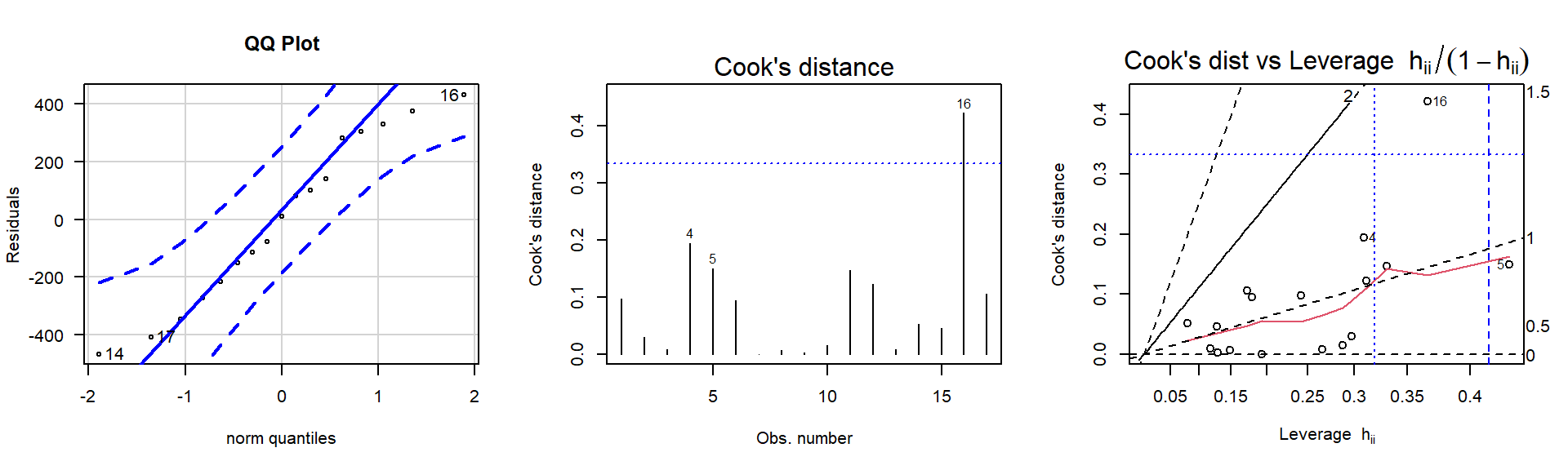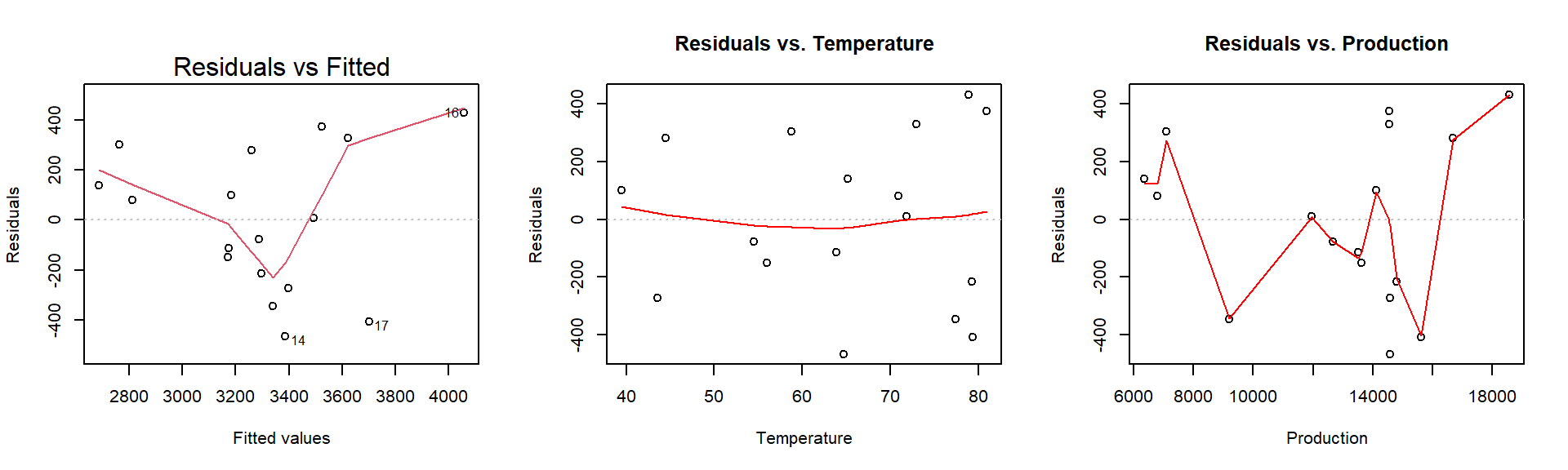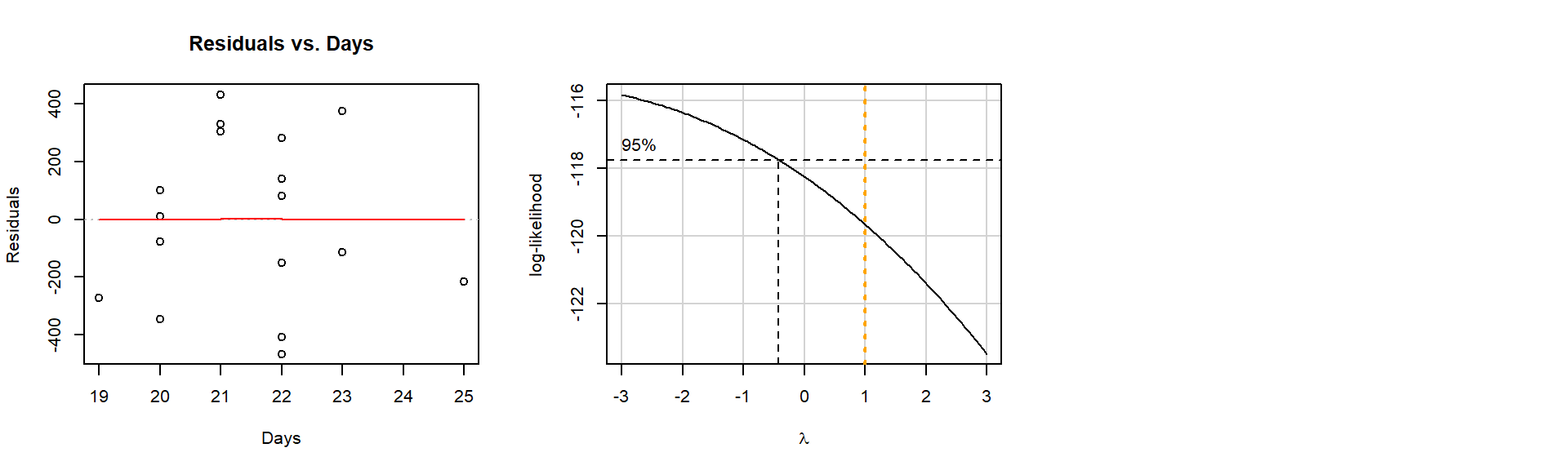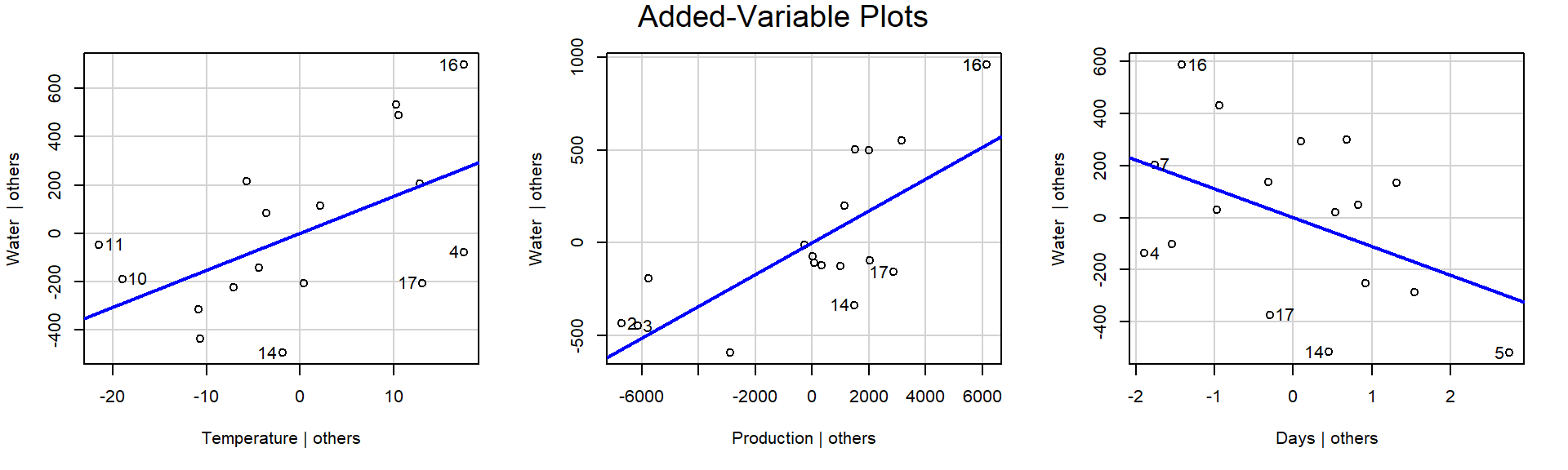### 3.2.1 Solution

From the diagnostic plots above,

## 3.3(1 p) Added variable plots

Use partial regression residual plots (added variable plots) to check for the need for transformations. If linearity is not supported, address and restart at the beginning.

## 3.4(1 p) Multiple regression hypothesis tests

State the hypothesis test and conclusion for each regression coefficient.

# use summary() to get t-tests of parameters (slope, intercept)
summary(lm_w_tpd)

Call:
lm(formula = Water ~ Temperature + Production + Days, data = dat_water)

Residuals:
Min      1Q  Median      3Q     Max
-465.05 -214.18    8.88  280.38  429.54

Coefficients:
Estimate Std. Error t value Pr(>|t|)
(Intercept) 3580.32788 1182.00657   3.029  0.00968 **
Temperature   15.23395    6.52782   2.334  0.03631 *
Production     0.08615    0.02261   3.810  0.00217 **
Days        -110.66108   60.61280  -1.826  0.09095 .
---
Signif. codes:  0 '***' 0.001 '**' 0.01 '*' 0.05 '.' 0.1 ' ' 1

Residual standard error: 316.2 on 13 degrees of freedom
Multiple R-squared:  0.5929,    Adjusted R-squared:  0.4989
F-statistic:  6.31 on 3 and 13 DF,  p-value: 0.0071

### 3.4.1 Solution

(I’ll get you started by writing the beginning of the first conclusion.)

Each hypothesis is testing, conditional on all other predictors being in the model, whether the addition of the predictor being tested explains significantly more variability in Water than without it.

For $$H_0: \beta_{\textrm{Temperature}}=0$$, the $$t$$-statistic is 2.334 with an associated p-value of 0.03631. Thus, we [reject / FAIL TO reject] $$H_0$$ concluding that the slope is [NOT] statistically significantly different from 0 conditional on the other variables in the model.

Similarly, for $$H_0: \beta_{\textrm{Production}}=0$$, the $$t$$-statistic is …

Similarly, for $$H_0: \beta_{\textrm{Days}}=0$$, the $$t$$-statistic is …

## 3.5(1 p) Multiple regression interpret coefficients

Interpret the significant coefficients of the multiple regression model.

### 3.5.1 Solution

(I’ll get you started by writing the beginning of the first conclusion.)

The coefficient for Temperature is estimated at $$\hat{\beta}_{\textrm{Temperature}}$$=15.23, thus we expect the Water to increase by 15.23 for each year increase in Temperature holding the other variables constant.

The coefficient for Production is estimated at …

The coefficient for Days is estimated at …

## 3.6(1 p) Multiple regression $$R^2$$

Interpret the Multiple R-squared value.

## 3.7(1 p) Summary

Summarize your findings in one sentence.

# 4 Unused plots

## Aside: While I generally recommend against 3D plots for a variety of reasons,
## so you can visualize the surface fit in 3D, here's a 3D version of the plot.
## I will point out a feature in this plot that we would't see in other plots
## and would typically only be detected by careful consideration
## of a "more complicated" second-order model that includes curvature.

# library(rgl)
# library(car)
# scatter3d(Water ~ Temperature + Production, data = dat_water)

These bivariate plots can help show the relationships between the response and predictor variables and identify each observation.